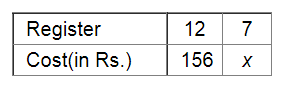# Rohit bought 12 registers for Rs 156, find the cost of 7 such registers.`
Question:

Rohit bought 12 registers for Rs 156, find the cost of 7 such registers.

Solution:

Let the cost of 7 registers be​ Rs x.If he buys less number of registers, the cost will also be less.

Therefore, it is a direct variation.

We get:

$12: 7=156: x$

$\Rightarrow \frac{12}{7}=\frac{156}{x}$

Applying cross muliplication, we get:

$x=\frac{156 \times 7}{12}$

$=91$

Thus, the cost of 7 such registers will be Rs 91 .